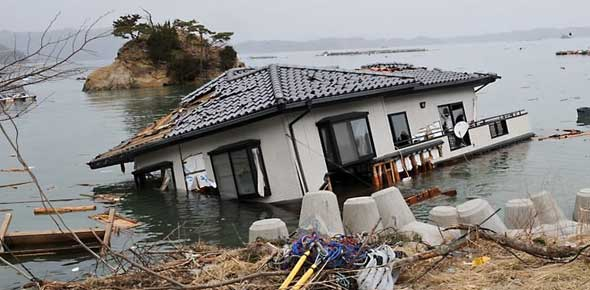# Earthquake Quiz For Kids

10 Questions | Attempts: 478
ShareSettings• 1.
Most earthquakes happen here : _______________________
• A.

The middle of the ocean

• B.

Plate boundaries

• C.

The middle of a plate

• D.

Everywhere

• 2.
Normal faults are created by this type of stress
• A.

Shear

• B.

Compression

• C.

Tension

• D.

Strike

• 3.
The Richter Scale measures ___________________ an earthquake.
• A.

The amount of damage done by

• B.

The number of people who felt

• C.

The number of occurrences of

• D.

The magnitude of

• 4.
Scientists use _______________________ to measure the strength of an earthquake.
• A.

People's observations

• B.

Seismographs

• C.

GPS

• D.

Tremor finders

• 5.
The Mercalli scale measures ______________________ an earthquake.
• A.

The strength of

• B.

The area covered by

• C.

The magnitude of

• D.

The amount of damage done by

• 6.
Scientists use _________________________ to find an earthquakes measurment on the Mercalli scale.
• A.

People's observations

• B.

Seismographs

• C.

Seismograms

• D.

GPS

• 7.
How many seismograms are needed to find the epicenter of an earthquake?
• A.

One

• B.

Two

• C.

Three

• D.

Four

• 8.
Which type of seismic waves move the fastest?
• A.

S Waves

• B.

P Waves

• C.

Surface Waves

• D.

They all travel the same speed

• 9.
Which type of seismic waves are the most destructive?
• A.

S Waves

• B.

P Waves

• C.

Surface Waves

• D.

Sub Surface Waves

• 10.
What does the amount of time between the arrival of the P Waves and the arrival of the S Waves tell a scientist?
• A.

How strong the earthquake was

• B.

The direction of the earthquake's focus

• C.

The magnitude of the earthquake

• D.

The distance the epicenter is from the seismograph

## Related TopicsBack to top
×

Wait!
Here's an interesting quiz for you.